You are here
Home > Class 11 > Relations and Functions Class 11

# Relations and Functions Class 11Chapter 2

### Ordered pair

A pair having an order is called ordered pair

Consider (a,b). a is called first component and b is called second component.

If  (a,b) = (c,d), the a = c and b = d

Eg : If (2x-3, 3y+1) = (5,7), find x and y

Ans:

Equating the first component

2x-3 = 5

2x = 5 + 3 = 8

x = 8/2 = 4

equating the second component

3y+1 = 7

3y = 7-1 = 6

y = 6/3 = 2

### Cross product (Cartesian Product)

Q. A × B is the set of all ordered pairs in which first element is from A and second element from B

1.  Let A = {1,2,3} and B = {4,5}, write A×B and B×A?

Ans:

A×B = {(1,4),(1,5),(2,4),(2,5),(3,4),(3,5)}

B×A = {(4,1),(4,2),(4,3),(5,1),(5,2),(5,3)}

Note :  If A = or B = ⌽-, then A×B =

n(A×B) = n(A).n(B)

n(A ) = 3, n(B) = 2

n(A×B) = 3×2 = 6

n(A×A) = n(A). n(A) = 3×3= 9

n(A×A×A) = n(A). n(A) .n(A)= 3×3×3= 27

Q. A = {-1,0}. Find A×A×A

Ans :

A×A = {-1,0}×{-1,0} = {(-1,-1), (-1,0), (0,-1), (0,0)}

A×A×A = {(-1,-1), (-1,0), (0,-1), (0,0)} ×{-1,0}

= {(-1,-1,-1), (-1,0,-1), (0,-1,-1), (0,0,-1), (-1,-1,1),

(-1,0,1), (0,-1,1), (0,0,1)}

Q. A = {1,2,3}, B = {3,4}, C = {4,5}, Verify that A×(BC)= (A×B)(A×C)

Ans :

L H S

BC = {4}

A×(BC) = {1,2,3}×{4}

= {(1,4), (2,4), (3,4)}

R H S

A×B = {1,2,3}×{3,4}

= {(1,3),(1,4),(2,3),(2,4),(3,3),(3,4)}

A×C = {1,2,3}×{4,5}

= {(1,4), (1,4),(2,4),(2,5),(3,4),(3,5)}

(A×B)(A×C) = {(1,4),(2,4),(3,4)}

L H S = R H S

Q.   A = {1,2,3,…14}. R is a relation on A defined by R = {(x,y) : 3x-y = 0}. Write R in roster form. Also write its domain, co domain and range?

Ans :

3x-y = 0

Put x = 1

3×1 – y = 0

3 – y = 0

Y = 3

Put x = 2, we get y = 6

Put x = 3, we get y = 9

Put x = 4, we get y = 12

Put x = 5, we get y = 15

R = {(1,3),(2,6),(3,9),(4,12)}

Domain = {1,2,3,4}

Range = {3,6,9,12}

Co domain = A

Q. Let A = {x,y,z}, B = {1,2} find the number of relations from A to B?

Ans:

m = 3, n= 2

Number of relations = 2mn

= 23×2 = 26 = 64

Q. A = {1,2,3}, B = {4,6,9}. R is a relation from A to B defined by

R = {(x,y) : the difference between x and y is odd}. Write R in roster form. Also write its domain, co domain and range?

Ans :

A×B = {(1,4), (1,6), (1,9), (2,4), (2,6), (2,9), (3,4),(3,6), (3,9)}

R = {(1,4), (1,6), (2,9), (3,4), (3,6)}

Domain = {1,2,3}

Range = {4,6,9}

Co domain = B

Q. A×A has 9 elements of which two elements are (-3,0) and (0,3). R is a Relation on A defined by R = {(x,y) : x + y = 0}. Write r in roster form

Ans :

A×A has 9 elements

A has 3 elements

A = {-3,0,3}

R = {(-3,3), (3,-3), (0,0)}

Q. A = {1,2,3,4}. R is a relation on A defined by R = {(a,b) : b is exactly divisible by a}. Write R in roster form

Ans :

A×A =  {(1,1), (1,2), (1,3), (1,4), (2,1), (2,2), (2,3), (2,4), (3,1), (3,2), (3,3), (3,4), (4,1), (4,2), (4,3), (4,4)}

R = {(1,1), (1,2), (1,3), (1,4), (2,2), (2,4), (3,3), (4,4) }

Q. The figure shows a relation from P to Q.

Write the relation in roster form and set builder formRelations and Functions Class 11

Ans :

Roster form

{(5,3), (6,4), (7,5)}

Set builder form

R = {(x,y) : x-y = 0, xP, yQ}

Functions

1. A relation from A to B is called a function if every element of A is related to unique element of B

2. Functions are generally denoted by f,g,h…

3.  F(x) = y means y is the image of x or x is the pre image of y

4.  Consider f :  A B . A is called domain and B is called co domain

5.  The set of elements in the co domain which have pre image is called range. Range is a subset of co domain

6. Number of relations from A to B is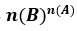7. If every horizontal line meets a graph only at a point, the graph represents a function.Fig 1 represents a function since every element in A has unique image in B

Fig 2 is not a function since 2 has more than one image and 3 has no image

Q. Find the domain and range of f(x) =x2 – 5x + 6 = 0

a =1, b = -5, c = 6Domain = R – {2,3}

Q. Find the domain and range of f(x) =x – 1 = 0
x = 1
Domain = R – {1}
f(x) = y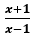= y

x+1 = y(x-1)
x+1 = xy – y
x-xy = -y -1
x(1-y) = -y-1
x =1-y = 0 ,  y = 1

Range = R – {1}

### Previous Years’ Questions Relations and Functions Class 11

1. f(x) = x2, g(x) = 2x + 1, find f+g, f-g, fg andf+g(x) = f(x)+g(x) = x2 + 2x+1
f-g(x) = f(x) – g(x) = x2-(2x+1)
fg(x) = f(x)g(x) = x2(2x+1)2. Find the domain and range of f(x) =X+1 ≥ 0
X≥-1
Domain= [-1, ∞)
Range   = [0, ∞)
3. Find the domain and range of f(x) =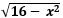Domain = [-4,4]
Range = [0,4]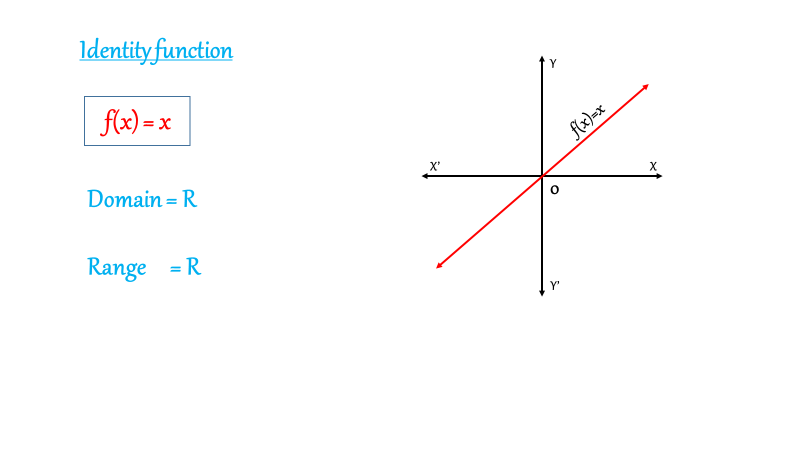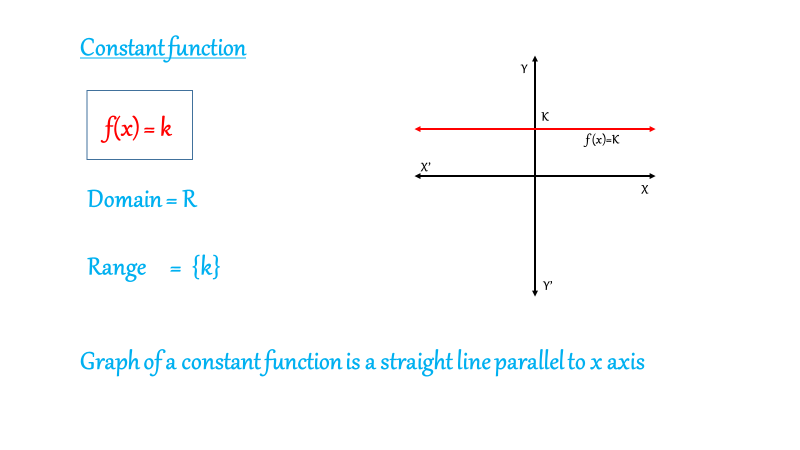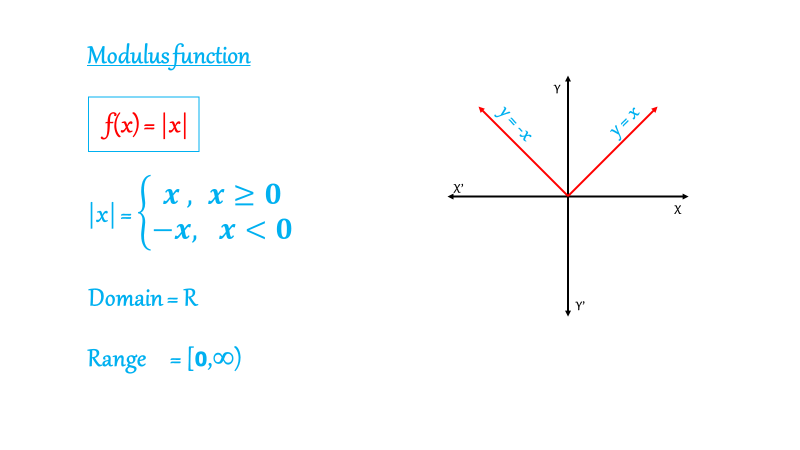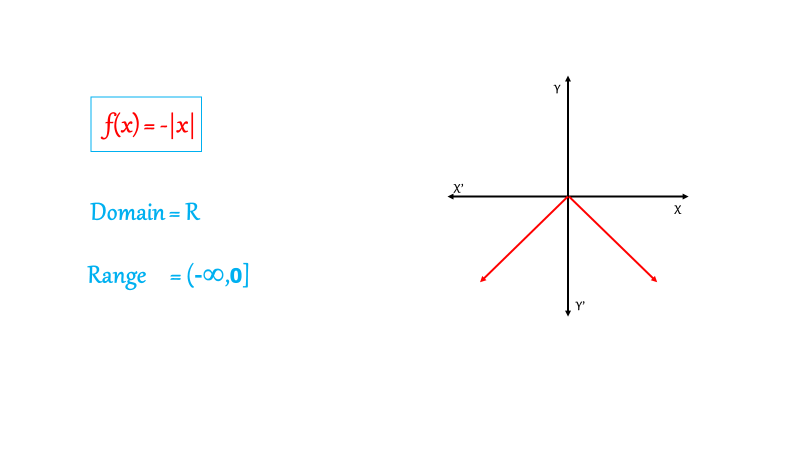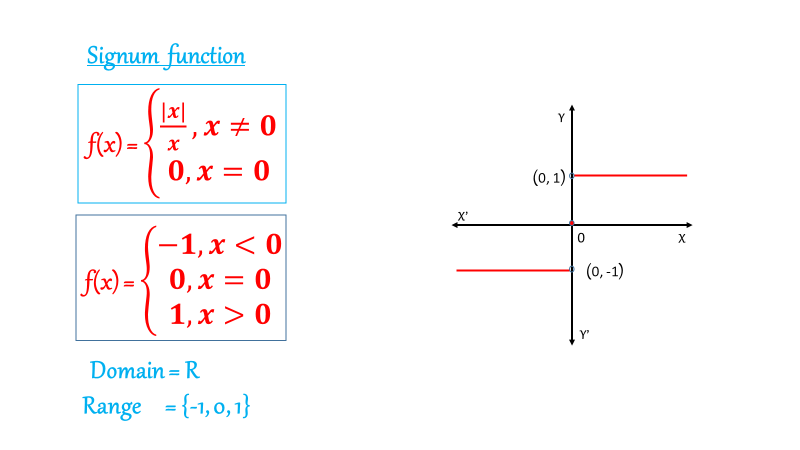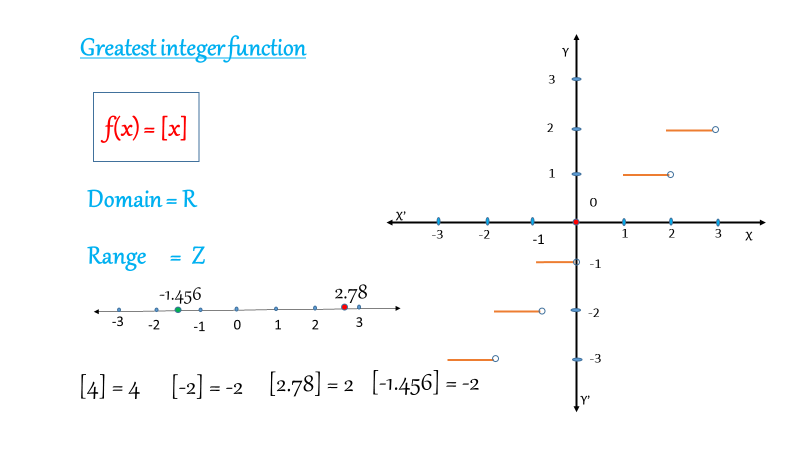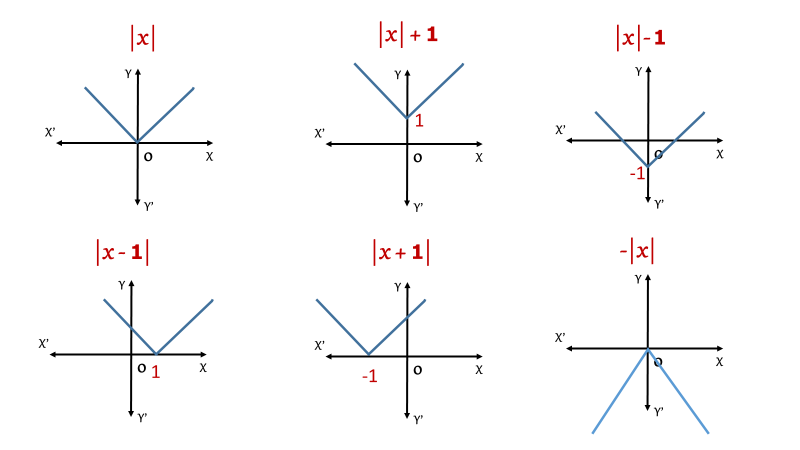### Exercise Relations and Functions Class 11

1.   A = {1,2,3}, B = {3,4}, find (A×B)(B×A)
2.  A = {-1,1}, find A × A × A
3.  Iffind x and y
4.  If n(AB) = 2, find n[(A×B)(B×A]
5.  A × A has 9 elements of which 2 elements are (-1,0) and (0,1). Find A×A.
6.  Write the domain and range of the relation R = {(x,x+5): x{0,1,2,3,4}}.
7.  R is a relation on N defined by R = {(x,y) : 2x + y = 10}. Write R in Roster form
8.  f(x) = x2, g(x) = 2x -1 , find f+g, f-g, fg and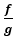9.  Find the domain and range of f(x) =10.Find the domain and range of f(x) =11. Find the domain and range of f(x) =12. Find the domain and range of f(x) =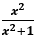13. Find the domain and range of f(x) =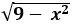14.  Let f = {(1,1), (2,3), (-1,-3)} be a linear function from Z into Z. fin f(x)
15.   Let f(x) = 2x + 3. Find the image of 3 and pre image of 1.